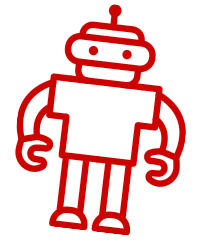## Math - Binary Number System

The number system most people are familiar with is called the decimal system. In math terms, it’s also known as “base 10”, and it works well because humans have ten fingers. In the decimal number system, 10 digits are used: 0, 1, 2, 3, 4, 5, 6, 7, 8, and 9. After 9, another digit is added to the end, becoming 10. And after 19 is 20, and so on.

There are also other number systems that exist, but they are not that well-known in real life. To computer science people, though, they know these by heart, especially the binary system. In binary, only two digits are used: 0 and 1. So in binary, 2 is “10”, 3 is “11”, 4 is “100”, and so on. Also, as with decimal numbers, binary numbers can also be added, subtracted, multiplied, and divided. Doing these operations is not just that intuitive, though, unlike the decimal numbers people are all used to.

To most people, this might be too complex to understand. But to computers, binary makes a lot of sense. Why? It’s because computer circuits are made up of tiny switches. Imagine a light switch: it has two possible states: on or off. This is very easy to represent in binary: 1 is for “on”, and 0 is for “off”.

Binary numbers are the language of computer circuits. This is how each of the components of computers talk to each other. This number system is the foundation of digital technology; it runs all of the gadgets of today.

The binary number system is taught more in computer science classes than mathematics ones, as the direct application of binary is really in the realm of computers. Some math classes may teach this concept just in passing. However, the more detailed lessons about binary and the logical operations that drive electronic circuits are mostly under computer science.